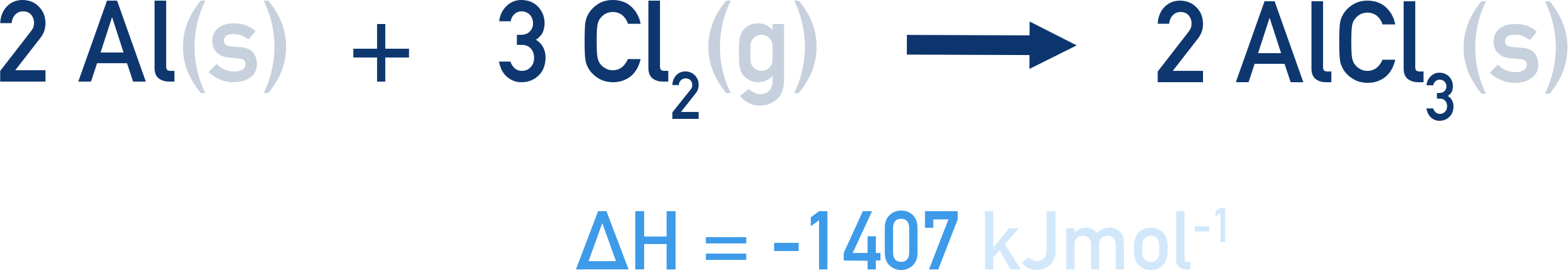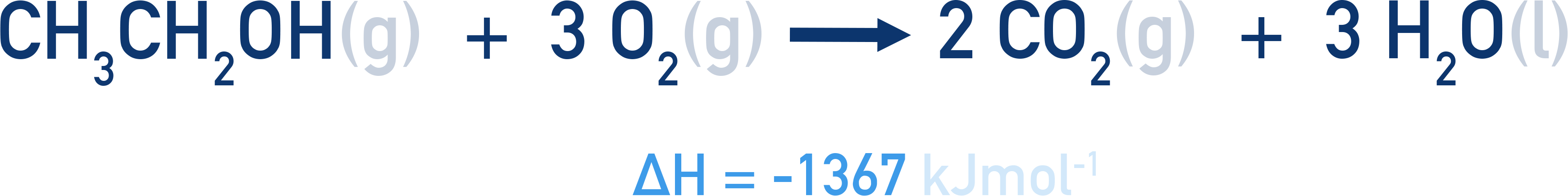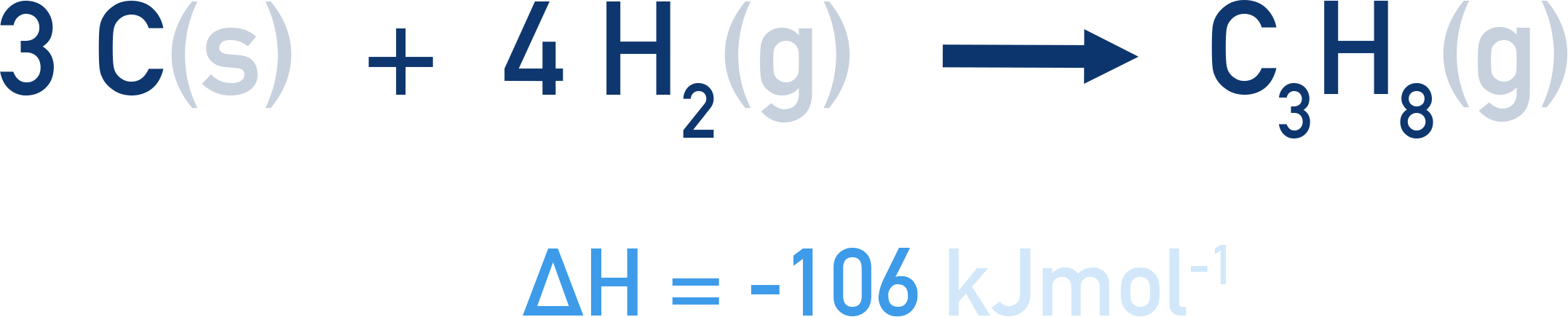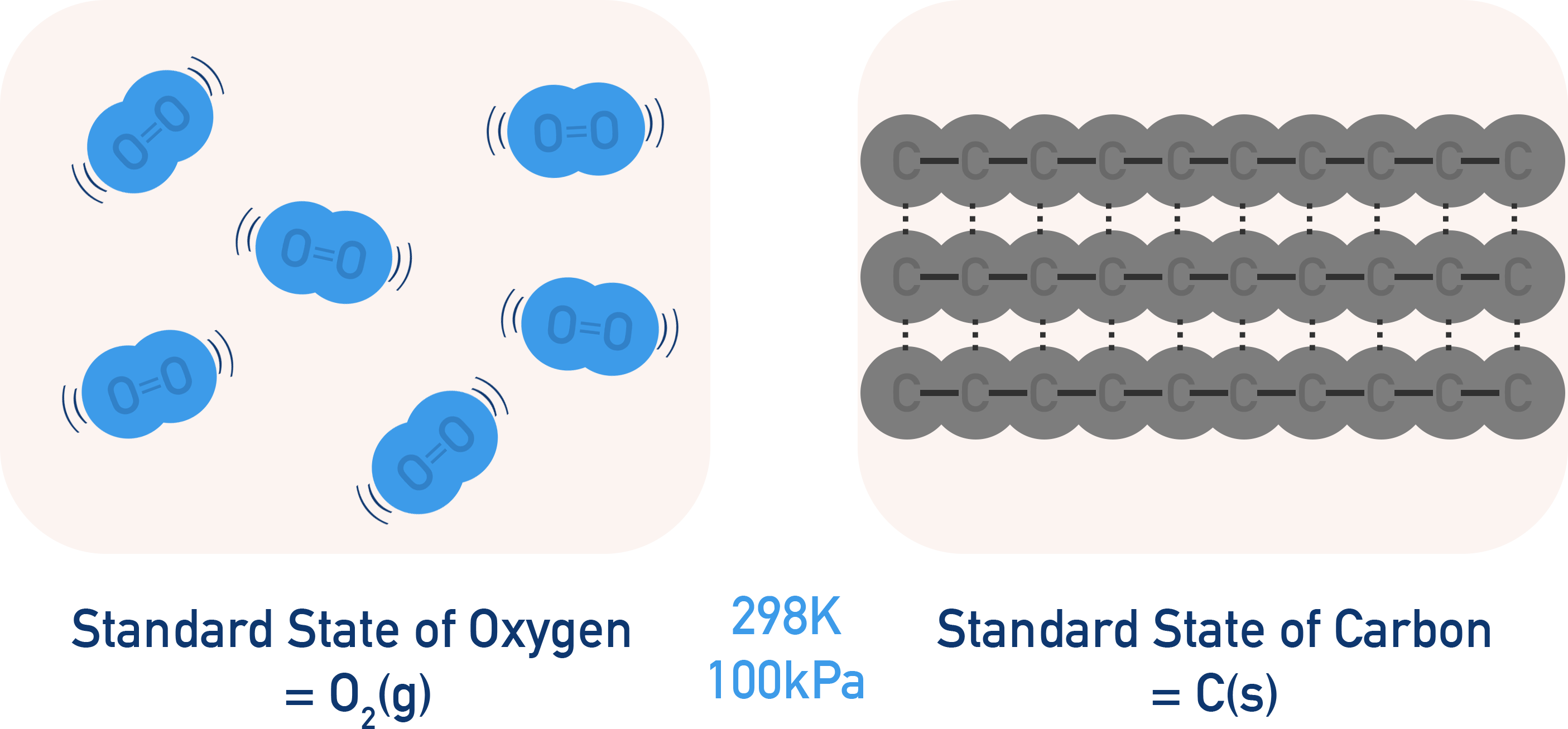Quick Notes Standard Enthalpy Changes

• Standard Enthalpy of Reaction, ΔHr
• Enthalpy change that occurs when reactants react in the molar quantities given in the reaction equation. Under standard conditions, reactants in their standard states.
• Standard Enthalpy of Combusion, ΔHc
• Enthalpy change that occurs when 1 mole of a substance is burned completely in oxygen. Under standard conditions, reactants in their standard states.
• Standard Enthalpy of Formation, ΔHf
• Enthalpy change that occurs when 1 mole of a substance is formed from its constituent elements. Under standard conditions, reactants in their standard states.
• Standard enthalpy changes are used to ensure enthalpy changes for different reactions can be compared and used in calculations.
• Standard conditions (298K and 100kPa) are used and reactants must be in their standard states (usual state at standard conditions).
• The ⦵ symbol is used to represent standard conditions and ΔH refers to a standard enthalpy change.
• Units are kJmol-1.

Full Notes Standard Enthalpy Changes

The conditions used when measuring enthalpy changes have to be the same every time if we want to compare and 'use' the enthalpy changes in calculations.

Such conditions are referred to as 'standard conditions' and are usually taken to be 298K and 100kPa. Reactants must also be in their standard states (see below). If an enthalpy change is measured under these conditions it is represented with the symbol ⦵ (ΔH).

There are lots of different types of reactions in chemistry but there are three common types of enthalpy change most students need to know at this level of study:

Standard Enthalpy of Reaction, ΔHr

Standard Enthalpy of Combustion, ΔHc

Standard Enthalpy of Formation, ΔHf

## Standard Enthalpy of Reaction

When reactants react in the molar amounts given in the equation for the reaction, the enthalpy change measured is an enthalpy of reaction. If standard conditions are used and reacants are in their standard states, the measured enthalpy change is the standard enthalpy of reaction.

Standard Enthalpy of Reaction, ΔHr: Enthalpy change that occurs when reactants react in the molar quantities given in the reaction equation. Under standard conditions, reactants in their standard states.

For Example...

Aluminium and chlorine can react together to form aluminium trichloride. The standard enthalpy change of reaction is -1407kJmol-1.This means that when two moles of aluminium reacts with three moles of chlorine, an enthalpy change of -1407 kJ occurs.

Standard enthalpies of reaction can be useful for determing enthalpy changes that occur when reagents are reacted in different molar amounts to those given by the reaction equation. For example, if four moles of aluminium reacted with six moles of chlorine, an enthalpy change of (2 x -1407) = -2814 kJ would occur.

## Standard Enthalpy of Combustion

When a substance is completely burnt in oxygen (combustion reaction), the enthalpy change measured is an enthalpy of combustion. If standard conditions are used and the substance being combusted and oxygen are in their standard states, the measured enthalpy change is the standard enthalpy of combustion (for the substance being combusted).

Standard Enthalpy of Combustion, ΔHc: Enthalpy change that occurs when one mole of a substance is burnt completely in oxygen. Under standard conditions, reactants in their standard states.

For Example...

Ethane can be completley burnt in oxygen to form carbon dioxide and water. The standard enthalpy change of combustion is -1367kJmol-1.This means that when one mole of ethanol reacts completley with oxygen (complete combustion), an enthalpy change of -1367 kJ occurs.

For standard enthalpies of combustion, it is only the moles of substance being combusted that matters - the moles of oxygen arn't really important. For example, if 0.5 moles moles of ethanol completely combusted, an enthalpy change of (0.5 x -1367) = -683.5 kJ would occur - we don't need to think about the moles of oxygen as complete combustion is occuring (the moles of oxygen present will always be in excess).

## Standard Enthalpy of Formation

When one mole of a susbtance is formed from its constituent elements, the enthalpy change measured is an enthalpy of formation. If standard conditions are used and the elements reacting are in their standard states, the measured enthalpy change is a standard enthalpy of formation.

Constituent elements refer to the elements that make up a compound. Water, H2O, for example, is made up hydrogen and oxygen, meaning its constituent elements are hydrogen and oxygen.

Standard Enthalpy of Formation, ΔHf: Enthalpy change that occurs when one mole of a substance is formed from its consituent elements (in their standard states) under standard conditions.

For Example...

The constituent elements of propane, C3H8, are carbon (C) and hydrogen (H). The standard enthalpy of formation for propane is -106kJmol-1.This means that when one mole of propane is formed from carbon and hydrogen (at standard conditions), an enthalpy change of -106 kJ occurs.

At standard conditions, the usual state of carbon is solid and hydrogen is a gas, H2. This means to form one mole of propane, C3H8, three moles of carbon and four moles of H2 gas are needed.

If two moles of propane gets formed, an enthalpy change of (2 x -106) = -212 kJ would occur.

Standard enthalpies of formation can be very hard to measure directly experimentally and are usually found indirectly using Hess's Law (See Hess's Law).

## What are Standard States?

Standard states refer to the usual state of an element or compound at standard conditions.

For example, at 298K and 100kPa, carbon will always be a solid. Meaning carbons standard state is solid. Equally, oxygen will always be a gas (made up of O2 molecules) at those conditions, meaning the standard state of oxygen is gas.The reason we have to refer to the 'usual' state is because some substances can exist in more than one state at 298K and 100kPa. Water, for example, is present as water vapour, H2O(g), in the air at those conditions as well as liquid form, H2O(l). It is far more likely for water to be a liquid at those conditions meaning its usual state would be liquid. As a result, the standard state of water is liquid, H2O(l).